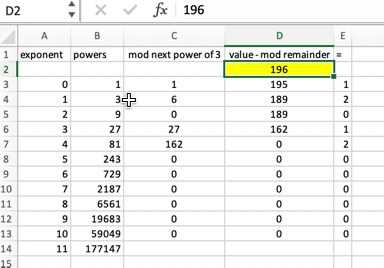# The ternary_out() Function

I believe my approach was okay for generating ternary (base 3) numbers, but for some reason I couldn’t get my algorithm to work. From last week’s Lesson, here’s what I tried:

1. Take a value n and determine the largest power divisible by n.
2. Subtract the power (or multiple thereof) from n.
3. Repeat the process on the remainder as you descend through the power table.

I just couldn’t get the thing to work, even after running it through the Code::Blocks debugger, fine-tuning, and other messin’ ’round.

Here’s the core of my code, the loop that processes a value stored in variable `n` with `tstring` as a char buffer to hold the output:

```/* build the return string */
for(x=0;x<10;x++)
{
if( n > powers[10-x] )
{
r = n / powers[10-x];
tstring[x] = r = '0';
n -= r * powers[10-x];
}
else
{
tstring[x] = '0';
}
}
tstring[x] = n + '0';
tstring = '\0';```

Don’t bother trying to analyze the thing; it didn’t work — and it has flaws, I admit. While it does concoct a valid ternary string for some numbers, it botches quite a few. Rather than debug and hammer the code further, I decided to solve the problem from the other direction: Start with the 30 digit’s position first and build the string backwards.

I know the basic methodology of writing a number in a specific counting base. Rather than write out all the permutations for base 3, I sought the assistance of Excel to process values. Figure 1 illustrates the worksheet I used and it reveals the formulas involved.Figure 1. I used this spreadsheet to help calculate values for my final ternary_out() function.

Once I got the spreadsheet working, it was a matter of translating the worksheet formulas into C. The results are shown below.

### 2020_06_13-Lesson-a.c

```#include <stdio.h>
#include <stdlib.h>

char *ternary_out(unsigned n)
{
static char tstring;
int powers;
int x,r;

/* check for overflow */
if( n<0 || n>65535 )
{
fprintf(stderr,"%d is out of range\n",n);
exit(1);
}

/* create the 3 powers table */
powers = 1;
for(x=1;x<12;x++)
{
powers[x] = powers[x-1]*3;
}

/* build the return string */
tstring = '\0';
for(x=0;x<11;x++)
{
r = n % powers[x+1];
n -= r;
tstring[10-x] = r/powers[x] + '0';
}

return(tstring);
}

int main()
{
unsigned t;

/* prompt for input */
printf("Enter a value: ");
scanf("%d",&t);

printf("%d in ternary is %s\n",t,ternary_out(t));

return(0);
}```

Here’s the heart of the ternary_out() function, which is far more elegant than what I worked on before:

`r = n % powers[x+1];`

Variable `n` is the original value, which is divided by a given power of three in the `powers[]` table. As an int type, variable `r` is is rounded down to the next integer value. This calculation is performed in column C in the worksheet (Figure 1).

`n -= r;`

The value of `r` is subtracted from the original value, `n`. This calculation is performed in the worksheet’s column D.

`tstring[10-x] = r/powers[x] + '0';`

Finally, the string’s next character, `tstring[10-x]`, is assigned the ASCII value of variable `r`. The math here is from column E in the worksheet. The result is the ternary digit in the proper position in array `tstring[]`.

Here’s a sample run:

```Enter a value: 1023 1023 in ternary is 0001101220```

I made one more improvement to the code, adding a second loop in the ternary_out() function to remove leading zeros from the string. Click here to view this update on my GitHub page.

Next week’s Lesson covers processing ternary input.